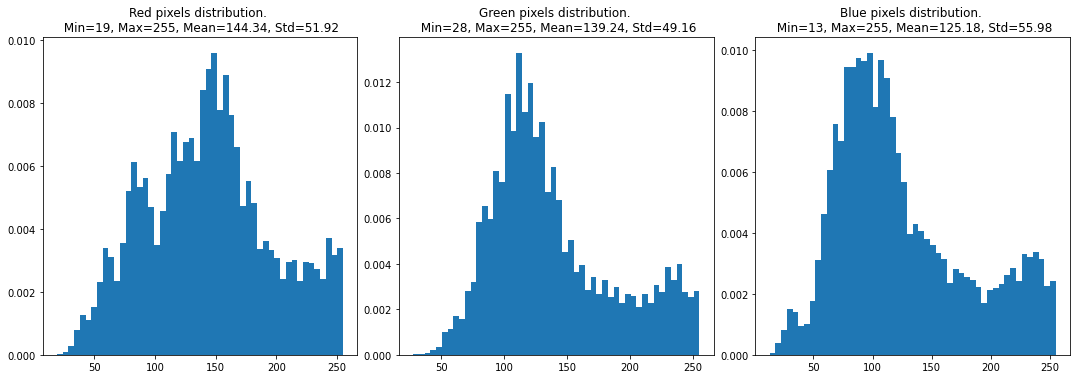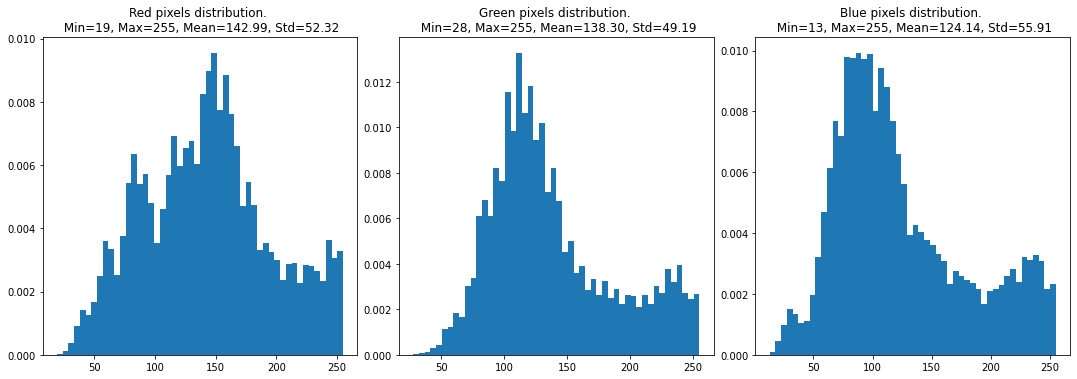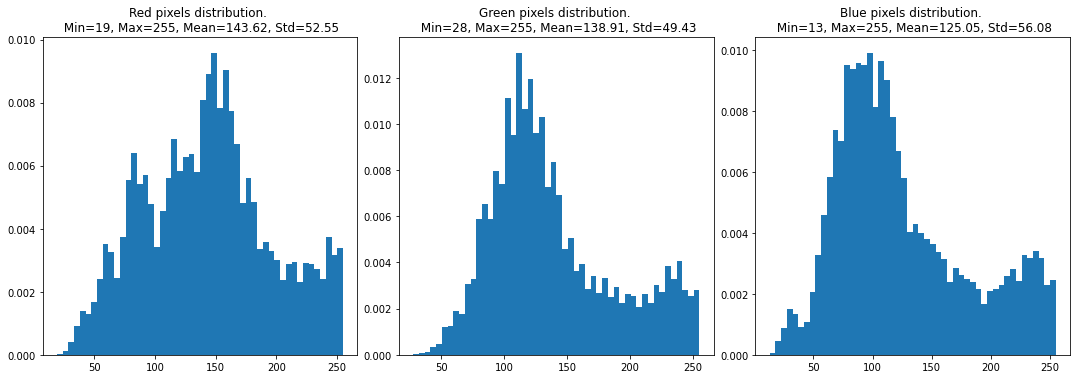# Apply the right Normalisation transformation for your data

Apply the right normalisation transformations to different datasets.

People usually get carried away with the main implementation and forget about the minimalistic pre-processing step. Many a time we copy the image transformation steps from Google. Yes, I know it happens, but there is a very simple way to know the normalization which is your dataset-specific. In this short notebook, you'll learn how to get the means and standard deviations of training, unlabelled, and validation datasets.Image normalization is one of the crucial thing to do in computer vision tasks. This notebook is rather a very small one that will give an short summary on different datasets.

# 1) Login to AIcrowd 🤩¶

In :
```#@title Login to AIcrowd
!pip install -U aicrowd-cli > /dev/null
```
```Please login here: https://api.aicrowd.com/auth/zo-wY53l5nP45gOH4OlcrGquu0Axok4c22AdzkgTWac
API Key valid
Saved details successfully!
```

# 2) Setup magically, run the below cell 😉¶

In :
```#@title Magic Box ⬛ { vertical-output: true, display-mode: "form" }
try:
import os
first_run = False
except:
first_run = True

if first_run:
%cd /content/
!mkdir -p data/
!mv *.tar.gz data/ && cd data && echo "Extracting dataset" && ls *.tar.gz | xargs -n1 -I{} bash -c "tar -xvf {} > /dev/null"

def run_pre_training_phase():
from run import ZEWDPCBaseRun
run = ZEWDPCBaseRun()
run.pre_training_phase = pre_training_phase
run.pre_training_phase(self=run, training_dataset=training_dataset)
# NOTE:It is critical that the checkpointing works in a self-contained way
#      As, the evaluators might choose to run the different phases separately.
run.save_checkpoint("/tmp/pretrainig_phase_checkpoint.pickle")

def run_purchase_phase():
from run import ZEWDPCBaseRun
run = ZEWDPCBaseRun()
run.pre_training_phase = pre_training_phase
run.purchase_phase = purchase_phase
# Hacky way to make it work in notebook
unlabelled_dataset.purchases = set()
run.purchase_phase(self=run, unlabelled_dataset=unlabelled_dataset, training_dataset=training_dataset, budget=3000)
run.save_checkpoint("/tmp/purchase_phase_checkpoint.pickle")
del run

def run_prediction_phase():
from run import ZEWDPCBaseRun
run = ZEWDPCBaseRun()
run.pre_training_phase = pre_training_phase
run.purchase_phase = purchase_phase
run.prediction_phase = prediction_phase
run.prediction_phase(self=run, test_dataset=val_dataset)
del run
```
```/content
fatal: destination path 'data-purchasing-challenge-2022-starter-kit' already exists and is not an empty directory.
Datasets for challenge #1024
┌───┬───────────────────┬──────────────────────────┬─────────┐
│ # │ Title             │ Description              │    Size │
├───┼───────────────────┼──────────────────────────┼─────────┤
│ 0 │ validation.tar.gz │ Validation dataset       │ 182 MiB │
│ 1 │ unlabelled.tar.gz │ Unlabelled image dataset │ 609 MiB │
│ 2 │ training.tar.gz   │ Training data            │ 304 MiB │
│ 3 │ debug.tar.gz      │ Debug dataset            │ 6.1 MiB │
└───┴───────────────────┴──────────────────────────┴─────────┘
validation.tar.gz: 100% 191M/191M [00:09<00:00, 19.6MB/s]
unlabelled.tar.gz: 100% 638M/638M [00:33<00:00, 19.3MB/s]
training.tar.gz: 100% 319M/319M [00:06<00:00, 51.0MB/s]
debug.tar.gz: 100% 6.43M/6.43M [00:00<00:00, 16.2MB/s]
Extracting dataset
```

In :
```from evaluator.dataset import ZEWDPCBaseDataset, ZEWDPCProtectedDataset
DATASET_SHUFFLE_SEED = 1022022

# Instantiate Training Dataset
training_dataset = ZEWDPCBaseDataset(
images_dir="./data/training/images",
labels_path="./data/training/labels.csv",
shuffle_seed=DATASET_SHUFFLE_SEED,
)
# Instantiate Unlabelled Dataset
unlabelled_dataset = ZEWDPCProtectedDataset(
images_dir="./data/unlabelled/images",
labels_path="./data/unlabelled/labels.csv",
budget=3000,  # Configurable Parameter
shuffle_seed=DATASET_SHUFFLE_SEED,
)
# Instantiate Validation Dataset
val_dataset = ZEWDPCBaseDataset(
images_dir="./data/validation/images",
labels_path="./data/validation/labels.csv",
drop_labels=True,
shuffle_seed=DATASET_SHUFFLE_SEED,
)
```
In :
```import numpy as np
import matplotlib.pyplot as plt

def show_dataset_summary(dataset):
images = []
for sample in dataset:
images.append(sample['image'])

images = np.array(images)
r_dim = images[:,:,:,0].ravel()
g_dim = images[:,:,:,1].ravel()
b_dim = images[:,:,:,2].ravel()

print("Dataset shape: ", images.shape)
fig, ax = plt.subplots(nrows=1, ncols=3, figsize=(15, 5))
fig.tight_layout()
ax.hist(r_dim, bins=50, density=True)
ax.set_title("Red pixels distribution. \n Min={}, Max={}, Mean={:.2f}, Std={:.2f}".format(r_dim.min(), r_dim.max(), r_dim.mean(), r_dim.std()))
ax.hist(g_dim, bins=50, density=True)
ax.set_title("Green pixels distribution. \n Min={}, Max={}, Mean={:.2f}, Std={:.2f}".format(g_dim.min(), g_dim.max(), g_dim.mean(), g_dim.std()))
ax.hist(b_dim, bins=50, density=True)
ax.set_title("Blue pixels distribution. \n Min={}, Max={}, Mean={:.2f}, Std={:.2f}".format(b_dim.min(), b_dim.max(), b_dim.mean(), b_dim.std()))
fig.show()
```
In :
```print("Summary for Training dataset")
show_dataset_summary(training_dataset)

print("Summary for Unlabelled dataset")
show_dataset_summary(unlabelled_dataset)

print("Summary for Validation dataset")
show_dataset_summary(val_dataset)
```
```Summary for Training dataset
Dataset shape:  (5000, 256, 256, 3)
Summary for Unlabelled dataset
Dataset shape:  (10000, 256, 256, 3)
Summary for Validation dataset
Dataset shape:  (3000, 256, 256, 3)
```Distribution of channel pixels among the datasets are nearly same. That is good thing

# Action points¶

Based on the above analysis, you can normalize your images for better performance with the below code snipped. For example the below normalization is given for training set.
NOTE: Don't get confused between the numbers shown in mean, std in graphs above and the numbers below. `ToTensor` normalize the image pixels by dividing each pixel with 255 and therefore you need to put the normalized means and standard deviations in `torchvision.transforms.Normalize`

``````transform = torchvision.transforms.Compose([
torchvision.transforms.ToTensor(),
torchvision.transforms.Normalize(
mean=[0.566, 0.546, 0.490],
std=[0.204, 0.193, 0.219],
),
])``````
1421